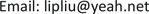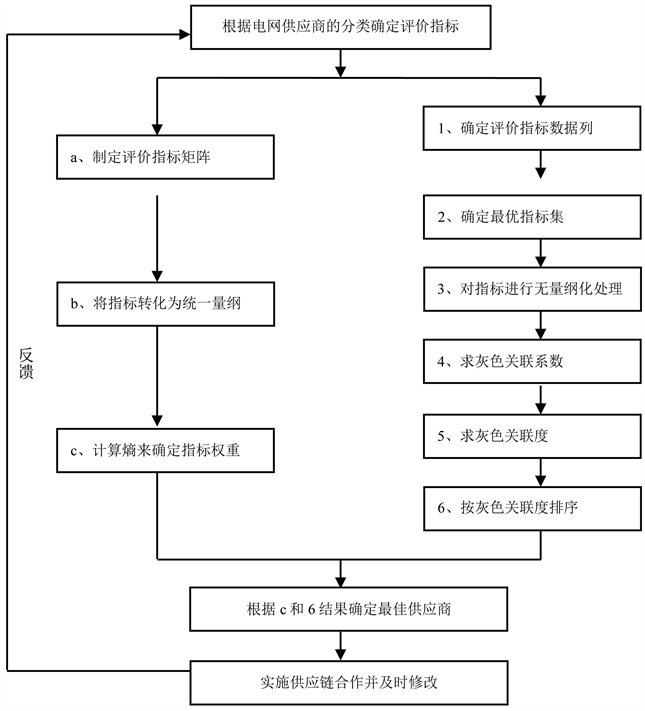﻿ 基于灰色关联分析的电力供应商评价模型研究 Electrical Supplier Evaluation Model Based on Gray Relational Analysis

Smart Grid
Vol.08 No.01(2018), Article ID:23933,6 pages
10.12677/SG.2018.81008

Electrical Supplier Evaluation Model Based on Gray Relational Analysis

Chengxian Wang

Jiangsu Electric Power Information Technology Co., Ltd., Nanjing JiangsuReceived: Feb. 7th, 2018; accepted: Feb. 20th, 2018; published: Feb. 28th, 2018ABSTRACT

According to the status and characteristics of power supplier evaluation, this paper establishes the evaluation method combining entropy weight coefficient method and gray relational analysis; the calculation procedure of supplier evaluation and selection is described. Finally, a case is given based on the real data, and the result of the case shows that the proposed method is effective for evaluating the suppliers.

Keywords:Supplier Evaluation, Entropy Weight Method, Gray Relational Analysis1. 引言

2. 电网供应商评价体系实现

2.1. 基于灰色关联分析的综合供应商评价步骤

2.1.1. 确定评价指标数据列

$\begin{array}{l}{X}_{1}=\left[{x}_{11},{x}_{12},\cdots ,{x}_{1n}\right]\\ {X}_{2}=\left[{x}_{21},{x}_{22},\cdots ,{x}_{2n}\right]\\ \text{\hspace{0.17em}}\text{\hspace{0.17em}}\text{\hspace{0.17em}}\text{\hspace{0.17em}}\text{\hspace{0.17em}}\text{\hspace{0.17em}}⋮\\ {X}_{m}=\left[{x}_{m1},{x}_{m2},\cdots ,{x}_{mn}\right]\end{array}$

2.1.2. 确定最优指标集

${X}^{*}=\left[{x}_{1}^{*},{x}_{2}^{*},\cdots ,{x}_{n}^{*}\right]$ ，其中 ${x}_{i}^{*}$ 为第i个指标的最优值，此最优值可根据诸方案中的最优值来确定(若某一指标取大值为好，则最优值取该指标在各方案中的最大值；若取小值为好，则最优值取该指标在各方案中的最小值)，选定最优指标集后，构造矩阵D：

$D=\left[\begin{array}{ccccc}{\text{x}}_{1}^{*}& {x}_{11}& {x}_{12}& \cdots & {x}_{1n}\\ {x}_{2}^{*}& {x}_{21}& {x}_{22}& \cdots & {x}_{2n}\\ ⋮& ⋮& ⋮& \ddots & ⋮\\ {x}_{m}^{*}& {x}_{m1}& {x}_{m2}& \cdots & {x}_{mn}\end{array}\right]$ (1)

2.1.3. 指标值进行规范化处理

$R=\left[\begin{array}{ccccc}{\text{r}}_{1}^{*}& {r}_{11}& {r}_{12}& \cdots & {r}_{1n}\\ {r}_{2}^{*}& {r}_{21}& {r}_{22}& \cdots & {r}_{2n}\\ ⋮& ⋮& ⋮& \ddots & ⋮\\ {r}_{m}^{*}& {r}_{m1}& {r}_{m2}& \cdots & {r}_{mn}\end{array}\right]$ (2)

2.1.4. 计算灰色关联度

${\epsilon }_{i}^{j}=\frac{\underset{j}{\mathrm{min}}\underset{i}{\mathrm{min}}|{r}_{i}^{*}-{r}_{ij}|+\rho \underset{j}{\mathrm{max}}\underset{i}{\mathrm{max}}|{r}_{i}^{*}-{r}_{ij}|}{|{r}_{i}^{*}-{r}_{ij}|+\rho \underset{j}{\mathrm{max}}\underset{i}{\mathrm{max}}|{r}_{i}^{*}-{r}_{ij}|}$ (3)

2.1.5. 基于灰色关联度进行供应商排序

3. 算例分析Figure 1. The procedure of electrical supplier evaluation

${w}_{i}=\left[0.0713,0.0418,0.0353,0.1132,0.1057,0.0744,0.0788,0.1345,0.2819,0.0631\right]$ .

4. 总结

Electrical Supplier Evaluation Model Based on Gray Relational Analysis[J]. 智能电网, 2018, 08(01): 70-75. http://dx.doi.org/10.12677/SG.2018.81008

1. 1. 陈金玉, 曾雪芳. 电网物资供应商分级管理策略及应用研究[J]. 电力与电工, 2013(4): 77-79.

2. 2. 陈春明. 供应商选择评价指标体系研究[J]. 学习与探索，2005(3): 197-201.

3. 3. 彭翔, 陈阳. 基于模糊层次分析法的电网物资供应商评价研究[J]. 商场现代化, 2010(28): 64-65.

4. 4. 马忠媛. 电力设备公开招标过程中供应商的选择研究[D]: [硕士学位论文]. 保定: 华北电力大学, 2007.

5. 5. 魏铁军. 电子商务环境下电网企业物资供应商评价与选择研究[D]: [硕士学位论文]. 北京: 华北电力大学, 2013.

6. 6. 宋吉昌, 郭珊珊, 刘春辉, 等. 基于主成分加权TOPSIS法的电网物资供应商评价研究[J]. 物流工程与管理, 2016, 38(6): 150-153.

7. 7. 曹媛. 考虑物流过程的电网物资供应商选择评价[D]: [硕士学位论文]. 北京: 华北电力大学(北京), 2014.